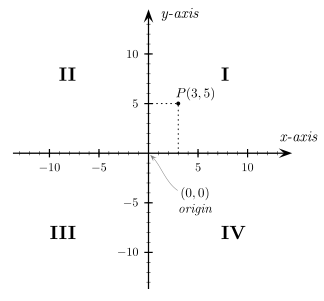# Cartesian coordinate system – 직각 좌표계In mathematics, the Cartesian coordinate system (also called rectangular coordinate system) is used to determine each point uniquely in a plane through two numbers, usually called the x-coordinate and the y-coordinate of the point. To define the coordinates, two perpendicular directed lines (the x-axis or abscissa and the y-axis or ordinate), are specified, as well as the unit length, which is marked off on the two axes (see Figure 1). Cartesian coordinate systems are also used in space (where three coordinates are used) and in higher dimensions.

Using the Cartesian coordinate system, geometric shapes (such as curves) can be described by algebraic equations, namely equations satisfied by the coordinates of the points lying on the shape.

Two-dimensional coordinate systemA Cartesian coordinate system in two dimensions is commonly defined by two axes, at right angles to each other, forming a plane (an xy-plane). The horizontal axis is normally labeled x, and the vertical axis is normally labeled y. In a three dimensional coordinate system, another axis, normally labeled z, is added, providing a third dimension of space measurement. The axes are commonly defined as mutually orthogonal to each other (each at a right angle to the other). (Early systems allowed “oblique” axes, that is, axes that did not meet at right angles, and such systems are occasionally used today, although mostly as theoretical exercises.) All the points in a Cartesian coordinate system taken together form a so-called Cartesian plane. Equations that use the Cartesian coordinate system are called Cartesian equations.

The point of intersection, where the axes meet, is called the origin normally labeled O. The x and y axes define a plane that is referred to as the xy plane. Given each axis, choose a unit length, and mark off each unit along the axis, forming a grid. To specify a particular point on a two dimensional coordinate system, indicate the x unit first (abscissa), followed by the y unit (ordinate) in the form (x,y), an ordered pair.

The choice of letters comes from a convention, to use the latter part of the alphabet to indicate unknown values. In contrast, the first part of the alphabet was used to designate known values.

An example of a point P on the system is indicated in Figure 3, using the coordinate (3,5).

The intersection of the two axes creates four regions, called quadrants, indicated by the Roman numerals I (+,+), II (−,+), III (−,−), and IV (+,−). Conventionally, the quadrants are labeled counter-clockwise starting from the upper right (“northeast”) quadrant. In the first quadrant, both coordinates are positive, in the second quadrant x-coordinates are negative and y-coordinates positive, in the third quadrant both coordinates are negative and in the fourth quadrant, x-coordinates are positive and y-coordinates negative (see table below.)

Three-dimensional coordinate systemThe three dimensional Cartesian coordinate system provides the three physical dimensions of space — length, width, and height. Figures 4 and 5, show two common ways of representing it. The three Cartesian axes defining the system are perpendicular to each other. The relevant coordinates are of the form (x,y,z). As an example, figure 4 shows two points plotted in a three-dimensional Cartesian coordinate system: P(3,0,5) and Q(−5,−5,7). The axes are depicted in a “world-coordinates” orientation with the z-axis pointing up. The x-, y-, and z-coordinates of a point can also be taken as the distances from the yz-plane, xz-plane, and xy-plane respectively. Figure 5 shows the distances of point P from the planes. The xy-, yz-, and xz-planes divide the three-dimensional space into eight subdivisions known as octants, similar to the quadrants of 2D space. While conventions have been established for the labelling of the four quadrants of the x-y plane, only the first octant of three dimensional space is labelled. It contains all of the points whose x, y, and z coordinates are positive. The z-coordinate is also called applicate.

출처: 위키 링크